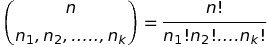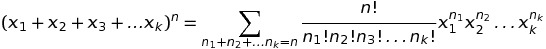# Multinomial Theorem

What is the multinomial coefficient?

The multinomial theorem provides an easy way to expand the power of a sum of variables. As “multinomial” is just another word for polynomial, this could also be called the polynomial theorem.

It tells us that when you expand any multinomial (x1+ x2 + ….xk)n the coefficients of every term x1n1 x2n2….xknk in the resulting polynomial will be:This is called the multinomial coefficient, and n1, n2,…., nk are integers which add up to make n.

SoThe exclamation mark signifies a factorial, where you multiply an integer by all the integers smaller than it. For example, 5! = 5 x 4 x 3 x 2 x 1 = 120.

The binomial theorem is a special case of the multinomial theorem.

## The Multinomial Theorem in Combinatorics

Suppose you have n distinct, differentiable items you are placing in k distinct groups. If you place n1 item group 1, n2 items in group two, and so on till you place nk items in the last group, the number of distinguishable permutations is given by the multinomial coefficient:The multinomial coefficient can also be used to find the number of distinguishable permutations of n objects when n= n1 + n 2 +….+nk and you have n1 items of kind 1, n2 items of type 2, and nk items of type k for every k. Here again the number of permutations is given by the multinomial formula (above).

## Examples

How many words can you make from the letters in mathematical? The word mathematical contains 2 m, 3 a, 2 t, one h, one e, one i, one c, and one l. Use the formula above, and you find that the number of permutations will be. Work the factorial, and you get 19958400. So there are 19958400 possible permutations of the letters in the word mathematical.

For another example, divide a class of 15 into groups of 3 for a final project. The groups are numbered a through e. There areways of divying up the class. Group the students randomly, and any student will have a 1 in 168168000 chance of ending up in any given group with any particular two friends.

## Sources

CITE THIS AS:
Stephanie Glen. "Multinomial Theorem" From StatisticsHowTo.com: Elementary Statistics for the rest of us! https://www.statisticshowto.com/multinomial-theorem/
---------------------------------------------------------------------------Need help with a homework or test question? With Chegg Study, you can get step-by-step solutions to your questions from an expert in the field. Your first 30 minutes with a Chegg tutor is free!